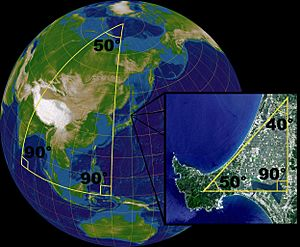# Non-Euclidean geometry facts for kids

Kids Encyclopedia FactsBehavior of lines with a common perpendicular in each of the three types of geometry

Non-Euclidean geometry is a type of geometry. Non-Euclidean geometry only uses some of the "postulates" (assumptions) that Euclidean geometry is based on. In normal geometry, parallel lines can never meet. In non-Euclidean geometry they can meet, either once (elliptic geometry), or infinitely many (hyperbolic geometry) times.

An example of Non-Euclidian geometry can be seen by drawing lines on a ball or other round object, straight lines that are parallel at the equator can meet at the poles

## OriginOn a sphere, the sum of the angles of a triangle is not equal to 180°. The surface of a sphere is not a Euclidean space, but locally the laws of the Euclidean geometry are good approximations. In a small triangle on the face of the earth, the sum of the angles is very nearly 180°.

It is called "Non-Euclidean" because it is different from Euclidean geometry, which was discovered by an Ancient Greek mathematician called Euclid. The different names for non-Euclidean geometries come from thinking of "straight" lines as curved lines, either curved inwards like an ellipse, or outwards like a hyperbola.

The first authors of non-Euclidean geometries were the Hungarian mathematician János Bolyai and the Russian mathematician Nikolai Ivanovich Lobachevsky, who separately published treatises on hyperbolic geometry around 1830. Others, such as Carl Friedrich Gauss, had earlier ideas, but did not publish their ideas at the time.Non-Euclidean geometry Facts for Kids. Kiddle Encyclopedia.